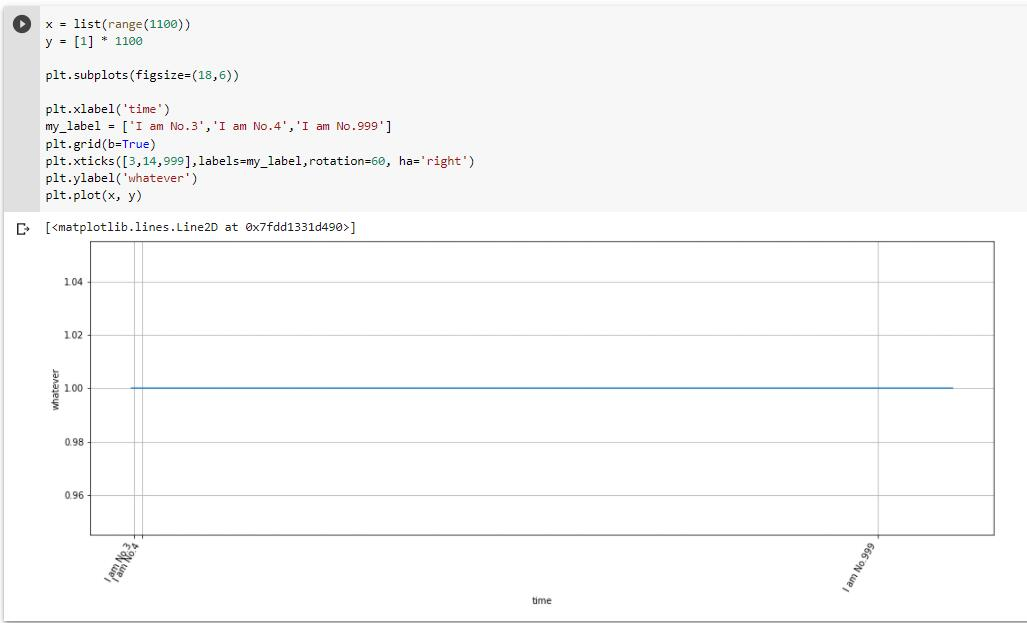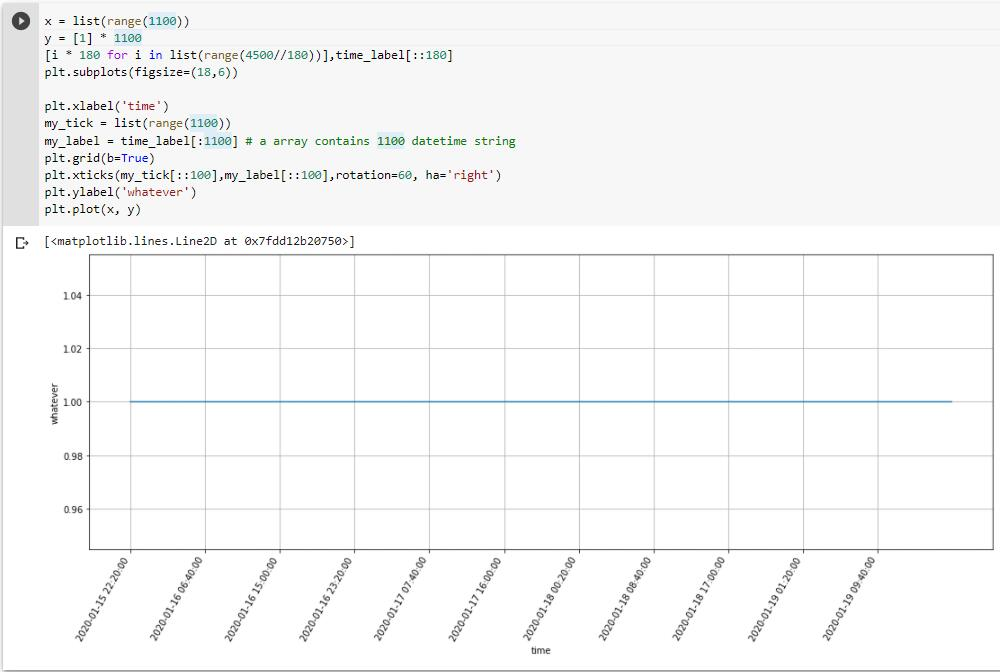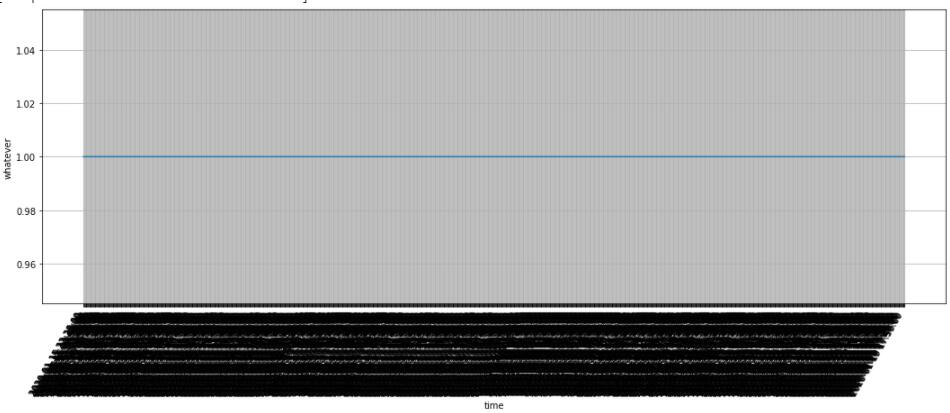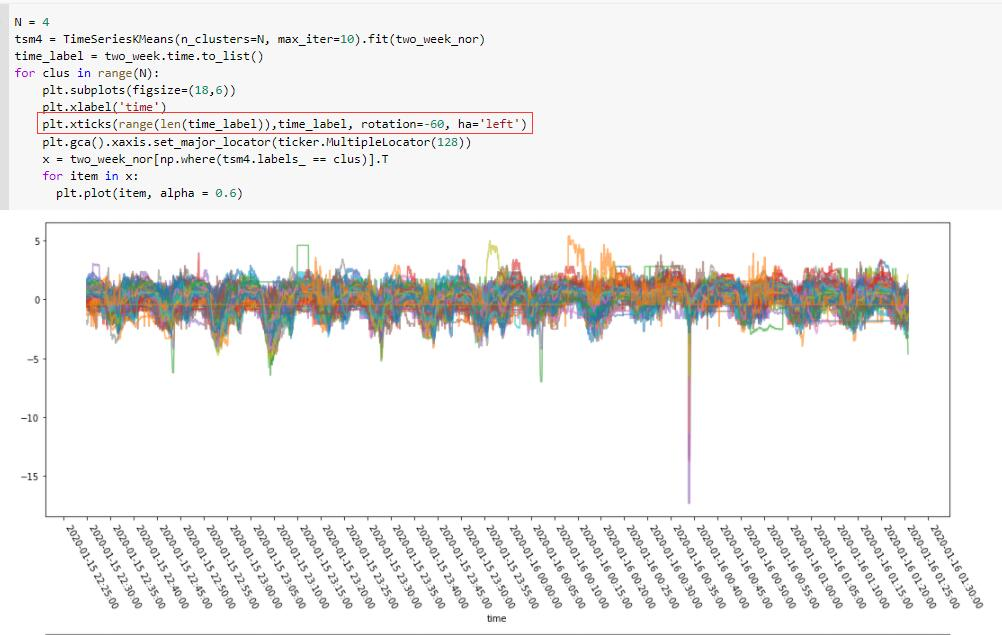# 如何使用Matplotlib.plot实现自定义横纵坐标轴标记（自定义标签与间隔放置）

Matplotlib是数据可视化中使用的各类绘图库中应用较为广泛的一个，近期使用时遇到大批量数据需要自定义坐标轴标记的需求，捣鼓了很久才搞明白，特此记录

### 如何自定义坐标

import Matplotlib.pyplot as plt
x = list(range(1100))
y =  * 1100

plt.subplots(figsize=(18,6))

plt.xlabel('time')                                      # 设置x坐标轴的名称
my_label = ['I am No.3','I am No.4','I am No.999']      # 自定义坐标轴值
plt.grid(b=True)                                        # 开启网格线
plt.xticks(ticks=[3,14,999],labels=my_label,
rotation=60, ha='right')                            # 自定义x轴内容
plt.ylabel('whatever')                                  # 设置y坐标轴的名称
plt.plot(x, y)                                          # 画图


plt.xticks(rotation=60, ha='right')中的rotation提供坐标标签旋转，旋转中心是标签的中心，所以旋转后可能出现看起来和坐标点对不齐的情况，这时候就需要使用ha参数选择标签的{'left','center','right'}边缘与坐标点对齐。### 间隔放置坐标轴标签的方法

import Matplotlib.pyplot as plt
x = list(range(1100))
y =  * 1100

plt.subplots(figsize=(18,6))

plt.xlabel('time')                         # 设置x坐标轴的名称
my_tick = list(range(1100))                # 可以用list(range(len(my_label))) 替代
my_label = time_label[:1100]               # 有1100个时间字符串的list
plt.grid(b=True)
plt.xticks(my_tick[::100],my_label[::100]
,rotation=60, ha='right')              # 利用切片来完成需求
plt.ylabel('whatever')
plt.plot(x, y)### 网上的另一种方法

N = 4
tsm4 = TimeSeriesKMeans(n_clusters=N, max_iter=10).fit(two_week_nor)
time_label = two_week.time.to_list()
for clus in range(N):
plt.subplots(figsize=(18,6))
plt.xlabel('time')
plt.xticks(range(len(time_label)),time_label, rotation=-60, ha='left')
plt.gca().xaxis.set_major_locator(ticker.MultipleLocator(128))
x = two_week_nor[np.where(tsm4.labels_ == clus)].T
for item in x:
plt.plot(item, alpha = 0.6)posted @ 2021-03-28 10:03  Approid  阅读(2556)  评论(0编辑  收藏  举报